JEE  >  Transpose of a Matrix

# Transpose of a Matrix | Mathematics (Maths) Class 12 - JEE

 1 Crore+ students have signed up on EduRev. Have you?

What is a matrix?

A matrix is a rectangular array of numbers or functions arranged in a fixed number of rows and columns. There are many types of matrices. Let us consider a matrix to understand more about them.
$A =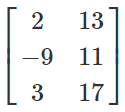_{3 \times 2}$
The above matrix A is of order 3 × 2. Thus, there are a total of 6 elements.
The horizontal array is known as rows and the vertical array are known as Columns.
Now, let us take another matrix.
$B =_{2 \times 3}$
The number of rows in matrix A is greater than the number of columns, such a matrix is called a Vertical matrix.
The number of columns in matrix B is greater than the number of rows. Such a matrix is called a Horizontal matrix.
One thing to notice here, if elements of A and B are listed, they are the same in number and each element which is there in A is there in B too. So, is A = B?
Before answering this, we should know how to decide the equality of the matrices.
A matrix P is said to be equal to matrix Q if their orders are the same and each corresponding element of P is equal to that of Q.
That is, if $P$ =$[p_{ij}]_{m×n}$ and $Q$ =$[q_{ij}]_{r×s}$ are two matrices such that$P$ = $Q$, then:

• m = r and n = s i.e. the orders of the two matrices must be same
• For every value of i and j, $p_{ij}$ = $q_{ij}$.

How to Find Transpose of a Matrix?
Let us now go back to our original matrices A and B. Though they have the same set of elements, are they equal?
The answer is no. That’s because their order is not the same. Now, there is an important observation. There can be many matrices which have exactly the same elements as A has.
Here, the number of rows and columns in A is equal to number of columns and rows in B respectively. Thus, the matrix B is known as the Transpose of the matrix A. The transpose of matrix A is represented by $A'$ or $A^T$. The following statement generalizes transpose of a matrix:
If $A$ = $[a_{ij}]_{m×n}$, then $A'$ =$[a_{ij}]_{n×m}$.
Thus Transpose of a Matrix is defined as “A Matrix which is formed by turning all the rows of a given matrix into columns and vice-versa.”

Let’s Work Out-

Example- Find the transpose of the given matrix
$M =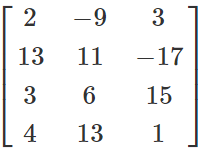$
Solution- Given a matrix of the order 4×3.
Transpose of a matrix is given by interchanging of rows and columns.
$M^T =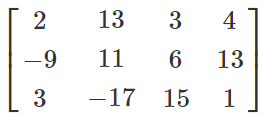$

Properties of Transpose of a Matrix

To understand the properties of transpose matrix, we will take two matrices A and B which have equal order. Some properties of transpose of a matrix are given below:

(i) Transpose of the Transpose Matrix
If we take transpose of transpose matrix, the matrix obtained is equal to the original matrix. Hence, for a matrix A, $(A’ )'$ = $A$
What basically happens, is that any element of A, i.e. $a_{ij}$ gets converted to $a_{ji}$ if transpose of A is taken. So, taking transpose again, it gets converted to $a_{ij}$, which was the original matrix $A$.

Example: If $N =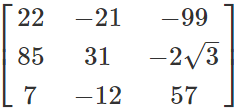$,
Then $N’ =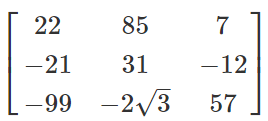$
Now, $(N’)'$ = $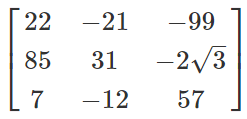$
= $N$

Transpose of an addition of two matrices A and B obtained will be exactly equal to the sum of transpose of individual matrix A and B.
This means,
$(A+B)'$ = $A’+B'$
Example-  If$P$ = $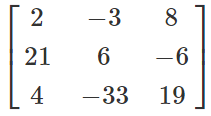$
and $Q$ = $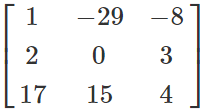$
$P + Q$ = $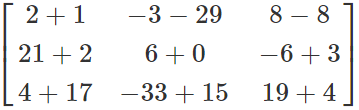$$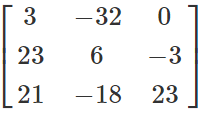$
$(P+Q)'$ = $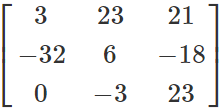$
$P’+Q'$ = $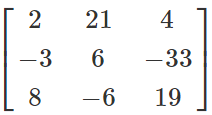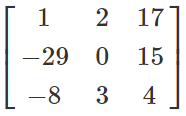$ = $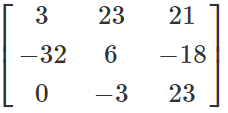$ = $(P+Q)'$
So, we can observe that $(P+Q)'$ = $P’+Q'$.

(iii) Multiplication by Constant
If a matrix is multiplied by a constant and its transpose is taken, then the matrix obtained is equal to transpose of original matrix multiplied by that constant. That is,
$(kA)'$ = $kA'$, where k is a constant
Example- If $P$ = $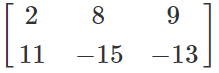_{2×3}$ and k is a constant, then $(kP)'$=
$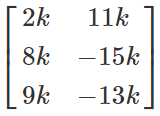_{2×3}$
$kP'$= $k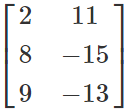_{2×3}$ = $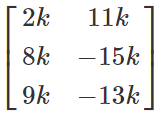_{2×3}$ = $(kP)'$
We can observe that $(kP)'$ = $kP'$.

(iv) Multiplication Property of Transpose
Transpose of the product of two matrices is equal to the product of transpose of the two matrices in reverse order. That is
$(AB)'$ = $B’A'$
Example: $A$= $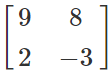$ and $B$ = $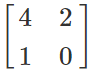$
Let us find $A×B$.
$A×B$ = $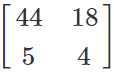\Rightarrow (AB)’ =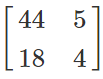$
$B’A'$ = $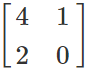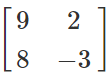$
= $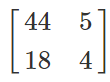$ = $(AB)'$
$(AB)'$ = $B’A'$
$A’B'$ = $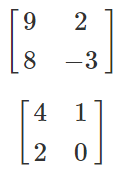=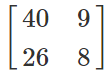$
We can clearly observe from here that (AB)’≠A’B’.
Those were properties of matrix transpose which are used to prove several theorems related to matrices.

The document Transpose of a Matrix | Mathematics (Maths) Class 12 - JEE is a part of the JEE Course Mathematics (Maths) Class 12.
All you need of JEE at this link: JEE

## Mathematics (Maths) Class 12

209 videos|218 docs|139 tests

## Mathematics (Maths) Class 12

209 videos|218 docs|139 tests

Track your progress, build streaks, highlight & save important lessons and more!(Scan QR code)

,

,

,

,

,

,

,

,

,

,

,

,

,

,

,

,

,

,

,

,

,

;